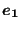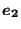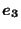### Distributing coupling loads

Distributing coupling loads are used if one wants to distribute a concentrated force and moment in a reference node across a complete surface. A typical application is the definition of a torque at the end of a (nearly) axisymmetric structure. You do not really know the force in each node, you just know the global value of the torque and the facial surface on which you would like to apply it. CalculiX calculates the corresponding forces in each node of the surface. For the theory the reader is referred to Section 6.7.3.

A distributed coupling is only active if a force was defined in the reference node (using a *CLOAD card) on a *COUPLING card in a direction defined underneath a *DISTRIBUTING card (which has to follow immediately underneath the *COUPLING card). The possible directions are 1 to 3 for forces (1 corresponds to a force along the x-axis etc.; this axis is local if the ORIENTATION parameter was used on the *COUPLING card, else it is global) and 4 to 6 for moments (4 corresponds to a moment about the x-axis etc.; this axis is local if the ORIENTATION parameter was used on the *COUPLING card, else it is global).

As soon as a *COUPLING card is detected followed by a *DISTRIBUTING card, the coupling surface is analyzed, its center of gravity is determined using area-defined weights and the constraints expressing the force in a given global direction in a concrete node of the coupling surface are stored in field coeffc(0..6,*). For force constraint i the information is stored as follows:

• coeffc(0,i): node and global degree of freedom (dof) to which this constraint applies in the form 10*(node-1)+dof
• coeffc(1,i): force in that node and direction for a unit force in local x-direction in the reference node
• coeffc(2,i): force in that node and direction for a unit force in local y-direction in the reference node
• coeffc(3,i): force in that node and direction for a unit force in local z-direction in the reference node
• coeffc(4,i): force in that node and direction for a unit moment about the local x-direction in the reference node
• coeffc(5,i): force in that node and direction for a unit moment about the local y-direction in the reference node
• coeffc(6,i): force in that node and direction for a unit moment about the local z-direction in the reference node

The number of such constraints (which may correspond to several distributing coupling definitions, indeed, the user may define more than one *COUPLING card followed by a *DISTRIBUTING card) is nfc. They are stored at the beginning of the calculation and may be addressed in any step.

In addition, for each degree of freedom defined underneath the *DISTRIBUTING card an entry in fields ikdc(*) and edc(12,*) is generated. Notice that the translational degrees of freedom are always active, irrespective whether the user has selected them underneath the *DISTRIBUTING card or not. The total number of distributing couplings is ndc. Each distributed coupling i corresponds to a (local or global depending on the presence of parameter ORIENTATION on the *COUPLING card) reference direction in the reference node and is stored in ikdc(i) in the form 8*(refnode-1)+refdir. This field is sorted in ascending order. The corresponding entries edc(1..12,i) correspond to:

• 1: the first force constraint in field coeffc for this distributing coupling +0.5
• 2: the last force constraint + 0.5
• 3: the number of the orientation on the *COUPLING card, if any (default 0) +0.5
• 4-6: local unit vectorof the coupling surface (in-surface)
• 7-9: local unit vectorof the coupling surface (in-surface)
• 10-12: local unit vectorof the coupling surface (orthogonal to the surface)

Also this information is stored at the beginning of the calculation.

Now, for each definition of a concentrated load in a node and direction, a check is performed by using field ikdc whether this node and direction correspond to a reference node an direction of a distributing coupling. If so, field edc indicates which force constraints are to be used and whether the force has to be projected because an orientation was defined. So at the time of reading the concentrated loads (in cloads.f) all point loads are calculated in the nodes of the coupling surface. Notice that the same rules apply as for *CLOAD: at the first occurrence in a step any previous load in that node and direction is replaced, at all further occurrences within the same step the value are added. It is not recommended to apply concentrated loads in any node belonging to a coupling surface apart from the ones by applying forces in the reference node.